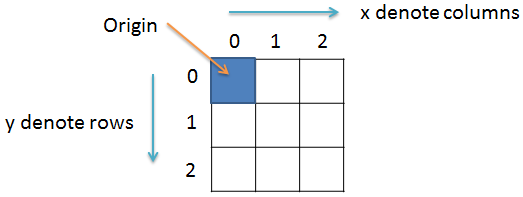# How to create a random pixel image in Java

Image Processing Project

In this project we will learn to create a random pixel image using Java programming language.

### Prerequisite

It is assumed that you have completed the projects titled How to read and write image file in Java and How to get and set pixel value in Java before starting this project.

### Color image to random pixel image

Creating a random image is based on random numbers. We have to perform the 3 steps to get the random pixel image.

1. Set the dimension of the image.
For this project we will take Width = 640 and Height = 320

2. Create a BufferedImage object img
BufferedImage img = new BufferedImage(width, height, BufferedImage.TYPE_INT_ARGB);

3. Generate random integer value for Alpha, Red, Green and Blue component and compose random pixels.

### Import the required classes

Create a new file and save it by the name RandomImage.java. Open the file and import the following:

``import java.io.File;``import java.io.IOException;``import java.awt.image.BufferedImage;``import javax.imageio.ImageIO;``

### Create the class

Next we will create the class by the name RandomImage and inside the class we will have the main() method. For this we will write:

``public class RandomImage{``  public static void main(String args[])throws IOException{``  // some code goes here...  }//main() ends here}//class ends here``

### Create BufferedImage

To create a random image we first have to create a BufferedImage object img and set its dimensions to 640x320. And we will create a File object f and set it to null. So, we will write the following code:

``//image dimension``int width = 640;``int height = 320;``//create buffered image object img``BufferedImage img = new BufferedImage(width, height, BufferedImage.TYPE_INT_ARGB);``//file object``File f = null;``

### Random pixel image code

We know that an image is made up of pixels which we can represent in 2D co-ordinate. So, we will create two variables x and y and use two for loops to traverse each pixels.For this we will write:

``for(int y = 0; y < height; y++){``  for(int x = 0; x < width; x++){  // some code goes here...  }}``

### Generate random pixel

In Java, to generate a random number we will use the random() method of the Math class. This generates a value greater than or equal to 0 and less less than 1. That is, we will get values like, 0.0 or 0.1 or 0.99999 etc. But we will never get 1.

We know that Alpha, Red, Green and Blue can take any integer value from 0 to 255. So, to get a value in the range 0 to 255 we will first multiply the random number with 256 and then convert the result to integer.

So, to get the random number we will write, Math.random()*256 which will give us a value greater than or equal to 0 and less than 256. Something like 0 or 123.45 or 255.999999. It will never give us 256.

Now to get the integer value we will type cast the floating value to int data type. For this we will write:

(int)(Math.random()*256)

So, in order to generate random Alpha, Red, Green and Blue value we will write:

``int a = (int)(Math.random()*256); //alpha``int r = (int)(Math.random()*256); //red``int g = (int)(Math.random()*256); //green``int b = (int)(Math.random()*256); //blue``

### Set new pixel value

Now, we will set the new pixel value. For this we will write:

``p = (a<<24) | (r<<16) | (g<<8) | b;img.setRGB(x, y, p);``

### Write image

After the for loop ends we will write the image file. For this we will write the following code:

``try{``  f = new File("D:\\Image\\Output.jpg");``  ImageIO.write(img, "png", f);``}catch(IOException e){``  System.out.println(e);``}``

### Final code

``/**`` * File: RandomImage.java`` * `` * Description:`` * Create a random color image.`` * `` * @author Yusuf Shakeel`` * Date: 01-04-2014 tue`` */``import java.io.File;``import java.io.IOException;``import java.awt.image.BufferedImage;``import javax.imageio.ImageIO;``public class RandomImage{``  public static void main(String args[])throws IOException{``     //image dimension``     int width = 640;``     int height = 320;``     //create buffered image object img``     BufferedImage img = new BufferedImage(width, height, BufferedImage.TYPE_INT_ARGB);``     //file object``     File f = null;``     //create random image pixel by pixel``     for(int y = 0; y < height; y++){``       for(int x = 0; x < width; x++){``         int a = (int)(Math.random()*256); //alpha``         int r = (int)(Math.random()*256); //red``         int g = (int)(Math.random()*256); //green``         int b = (int)(Math.random()*256); //blue` `         int p = (a<<24) | (r<<16) | (g<<8) | b; //pixel` `         img.setRGB(x, y, p);``       }``     }``     //write image``     try{``       f = new File("D:\\Image\\Output.png");``       ImageIO.write(img, "png", f);``     }catch(IOException e){``       System.out.println("Error: " + e);``     }``  }//main() ends here``}//class ends here``